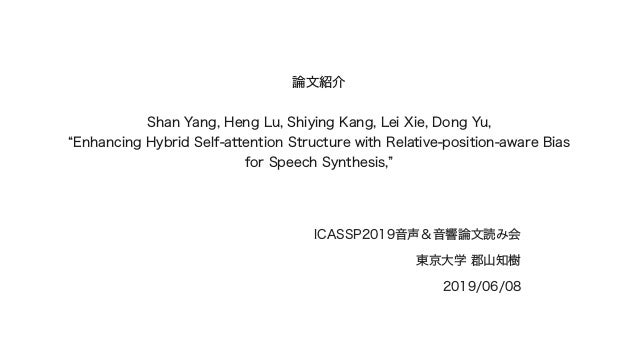Successfully reported this slideshow.Upcoming SlideShare
×

ICASSP2019音声＆音響論文読み会 論文紹介（合成系） #icassp2019jp

772 views

Published on

S. Yang, H. Lu, S. Kang, L. Xie and D. Yu, "Enhancing Hybrid Self-attention Structure with Relative-position-aware Bias for Speech Synthesis," Proc. ICASSP, pp. 6910-6914, 2019.

Published in: Technology
• Full Name
Comment goes here.

Are you sure you want to Yes No• Be the first to comment

ICASSP2019音声＆音響論文読み会 論文紹介（合成系） #icassp2019jp

1. 1.
2. 2. ‣ ‣
3. 3. ‣     Perceptron block Perceptron block Perceptron block GPR GPR GPR GPR GPR
4. 4.
5. 5. ‣ ‣
6. 6. ‣ ‣ ‣
7. 7.
8. 8. ‣   ‣ ‣
9. 9. αj = exp(ej) ∑ n k=1 exp(ek) y = n ∑ j=1 αjvj y αj vj kj q j Dej = q⊤ kj D v1, v2, …, vn k1, k2, …, kn q α1, α2, …, αn y
10. 10. eij = (WQ xi)⊤ (WK xj) D αij = exp(eij) ∑ n k=1 exp(eik) yi = n ∑ j=1 αijWV xj yi xi αij WV WK WQ i, j D x1, x2, …, xn y1, y2, …, yn {αij}
11. 11. ‣ ‣ ‣
12. 12.   (PE(k))d = sin ( k 10000 d D ) d sin ( k 10000 d D ) d eij = (WQ x′i)⊤ (WK x′j) D αij = exp(eij) ∑ n k=1 exp(eik) αij x′k = xk + PE(k)
13. 13. eij = (WQ xi)⊤ (WK xj + aK ij ) D αij = exp(eij) ∑ n k=1 exp(eik) aK ij   αij ω−2 ω−1 ω0 ω1 ω2
14. 14.
15. 15.
16. 16.
17. 17.
18. 18. https://syang1993.github.io/relation-aware/
19. 19. ‣     ‣ ‣ ‣
20. 20. ‣ ‣ ‣